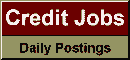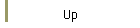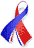DefaultRisk.com the web's biggest credit risk modeling resource.doi> search: A or B Export citation to:- HTML- Text (plain)- BibTeX- RIS- ReDIF

An Exponential Approximation to the Hockey Stick Function

by Ian Iscoe of Algorithmics, Inc.,
Ken Jackson of the University of Toronto,
Alex Kreinin of Algorithmics, Inc., and
Xiaofang Ma of the Bank of Montreal

March 19, 2010

Abstract: The hockey stick (HS) function plays an important role in pricing and risk management of many financial derivatives. This paper considers approximating the HS function by a sum of exponentials. This enables the efficient computation of an approximation to the expected value of the HS function applied to a sum of conditionally independent nonnegative random variables, a task that arises in pricing many financial derivatives, CDOs in particular. The algorithm proposed by Beylkin and Monzón is used to determine the parameters of the exponential approximation to the hockey stick function. Theoretical properties of the approximation are studied and numerical results are presented.

Keywords: Synthetic CDO, hockey stick function, exponential approximation.

Previously titled: "On Exponential Approximation to the Hockey Stick Function"

Books Referenced in this paper:  (what is this?)